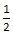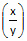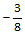# Number System & Theory & Number Properties

This topic is an important and will usually account for about a quarter of the number of questions that typically appear in any B school entrance test - be it TANCET or CAT or GMAT. Concepts tested include prime numbers, composite numbers, testing whether a given number is prime, co prime or relatively prime numbers, properties of perfect squares, properties of perfect cubes, LCM, HCF or GCD, remainders, test of divisibility, unit digits of numbers, factorials (likely to be tested in XAT than in TANCET), expressing numbers in different bases, number of factors of a positive integer, sum of factors of a positive integer, product of factors of a positive integers, and properties of indices.

1. If both 112 and 33 are factors of the number a * 43 * 62 * 1311, what is the smallest possible value of 'a'?

1. 121
2. 3267
3. 363
4. 33
5. 37

Concept: Divisors, Prime factors

2. Find the largest five digit number that is divisible by 7, 10, 15, 21 and 28.

1. 99,840
2. 99,900
3. 99,960
4. 99,990
5. 99,970

Concept: Test of divisibility

3. Anita had to multiply two positive integers. Instead of taking 35 as one of the multipliers, she incorrectly took 53. As a result, the product went up by 540. What is the new product?

1. 1050
2. 540
3. 1440
4. 1520
5. 1590

Concept: Word Problem

4. Let x, y and z be distinct integers. x and y are odd and positive, and z is even and positive. Which one of the following statements cannot be true?

1. (x - z)2 y is even
2. (x - z) y2 is odd
3. (x - z) y is odd
4. (x - y)2 z is even
5. (x + y)3 z is even

Concept: Operations on Odd & Even integers

5. When a number is divided by 36, it leaves a remainder of 19. What will be the remainder when the number is divided by 12?

1. 10
2. 7
3. 19
4. 192
5. None of these

Concept: Remainders

6. The sum of the first 100 natural numbers, 1 to 100 is divisible by ____

1. 2, 4 and 8
2. 2 and 4
3. 2
4. 100
5. None of these

Concept: Sum of AP and divisibility

7. How many different factors does 48 have, excluding 1 and 48?

1. 12
2. 4
3. 8
4. 10
5. None of these

Concept: Number of factors

8. 1025 - 7 is divisible by _____

1. 2
2. 3
3. 9
4. Both (2) and (3)

Concept: Test of divisibility

9. Find the G.C.D of 12x2y3z2, 18x3y2z4, and 24xy4z3

1. 6xy2z2
2. 6x3y4z3
3. 24xy2z2
4. 24xy3z2
5. 18x2y2z3

Concept: HCF or GCD

10. What is the value of M and N respectively? If M39048458N is divisible by 8 & 11; where M & N take integer values from 0 to 9, inclusive?

1. M = 7; N = 8
2. M = 8; N = 6
3. M = 6; N = 4
4. M = 5; N = 4
5. M = 4; N = 6

Concept: Test of divisibility

11. 48 students have to be seated such that each row has the same number of students as the others. If at least 3 students are to be seated per row and at least 2 rows have to be there, how many arrangements are possible?

1. 4
2. 10
3. 8
4. 7
5. 6

Concept: Counting Methods

12. If two fractions, each of which has a value between 0 and 1, are multiplied together, the product will be ___

1. always greater than either of the original fractions
2. always less than either of the original fractions
3. sometimes greater and sometimes less than either of the original fractions
4. remains the same
5. never less than either of the original fractions

Concept: Properties of fractions

13. Three Vice Presidents (VP) regularly visit the plant on different days. Due to labour unrest, VP (HR) regularly visits the plant after a gap of 2 days. VP (Operations) regularly visits the plant after a gap of 3 days. VP (sales) regularly visits the plant after a gap of 5 days. The VPs do not deviate from their individual schedules. CEO of the company meets the VPs when all the three VPs come to the plant together. CEO is on leave from January 5th to January 28th, 2012. Last time the CEO met the VPs on January 3, 2012. When is the next time the CEO will meet all the VPs?

1. February 6, 2012
2. February 7, 2012
3. February 8, 2012
4. February 9, 2012
5. None of the above

Concept: LCM & Common Multiples

14. How many divisors does 7200 have?

1. 20
2. 4
3. 54
4. 32

Concept: Number of factors

15. What is the highest power of 7 that will divide 5000! without leaving a remainder? (5000! means factorial 5000)

1. 4998
2. 714
3. 832
4. 816

Concept: Factorial

16. 'a' and 'b' are the lengths of the base and height of a right angled triangle whose hypotenuse is 'h'. If the values of 'a' and 'b' are positive integers, which of the following cannot be a value of the square of the hypotenuse?

1. 13
2. 23
3. 37
4. 41

Concept: Properties of perfect squares

17. If x, y, z are chosen from the three numbers, -3,and 2, what is the largest possible value of the expressionz2?

1.2. 16
3. 24
4. 36
5. 54

Concept: Number Properties

18. If ( 0.004 * 10a )( 0.32 * 10b ) = 128 * 103, a + b = ?

1.2. 3
3. 5
4. 6
5. 8
6. -2

Concept: Properties of indices

19. There are 35 steps in a temple. By the time Chithra comes down two steps, Madhu goes up one step. If they start simultaneously and keep their speed uniform, then at which step from the bottom will they meet?

1. 9th step
2. 12th step
3. 13th step
4. 8th step
5. None of the above

Concept: Relative Speed

20. If a, b, c and d are four different positive integers selected from 1 to 25, then the highest possible value of $$frac{$a + b$ + (c + d)}{(a + b) + (c - d)}$\$ would be:

1. 47
2. 49
3. 51
4. 96
5. None of the above

Concept: Properties of Fractions

21. The value of 6ab2c3 * 4b-2c-3d is

1. 6a
2. 4b
4. 4d
5. 0

Concept: Rules of Indices

## Online TANCET MBA CourseTry it Free!

Register in 2 easy steps and start learning in 5 minutes!